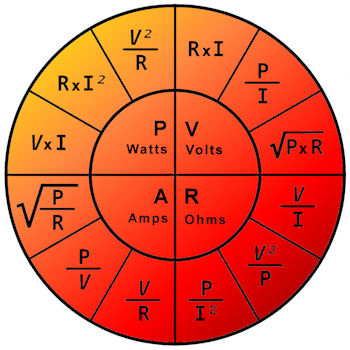# Ohms law calculator

The heaters we supply are almost all based on resistance wire. Silicone heaters, cartridgeheaters and furnaces have resistance wire in common.

Using Ohm's law, it is easy to calculate the power of a specific heater performing with a certain voltage. It will be even easier when you use our Ohms Law calculator below.

Add at least 2 values to get the 2 remaining values. Example: You have 230V available and need 800 Watts. After input in the calculator you will learn that 66 Ohms and almost 3.5 Amps are applicable for this heating system.

Spanning (V):
V

Stroom (I):
A

Weerstand (R):
Ω

Vermogen (P):
W

Ohm's law states that the current through a conductor between two points is directly proportional to the voltage across the two points. Introducing the constant of proportionality, the resistance, one arrives at the usual mathematical equation that describes this relationship.

V = I x R

where I is the current through the conductor, V is the voltage measured across the conductor and R is the resistance of the conductor. More specifically, Ohm's law states that the R in this relation is constant, independent of the current. If the resistance is not constant, the previous equation cannot be called Ohm's law, but it can still be used as a definition of static/DC resistance.Ohm's law is an empirical relation which accurately describes the conductivity of the vast majority of electrically conductive materials over many orders of magnitude of current.

The law was named after the German physicist Georg Ohm, who, in a treatise published in 1827, described measurements of applied voltage and current through simple electrical circuits containing various lengths of wire.

Source: Wikipedia